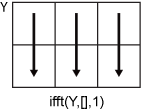# ifft

Inverse fast Fourier transform

## Syntax

``X = ifft(Y)``
``X = ifft(Y,n)``
``X = ifft(Y,n,dim)``
``X = ifft(___,symflag)``

## Description

example

````X = ifft(Y)` computes the inverse discrete Fourier transform of `Y` using a fast Fourier transform algorithm. `X` is the same size as `Y`. If `Y` is a vector, then `ifft(Y)` returns the inverse transform of the vector.If `Y` is a matrix, then `ifft(Y)` returns the inverse transform of each column of the matrix.If `Y` is a multidimensional array, then `ifft(Y)` treats the values along the first dimension whose size does not equal 1 as vectors and returns the inverse transform of each vector. ```

example

````X = ifft(Y,n)` returns the `n`-point inverse Fourier transform of `Y` by padding `Y` with trailing zeros to length `n`.```

example

````X = ifft(Y,n,dim)` returns the inverse Fourier transform along the dimension `dim`. For example, if `Y` is a matrix, then `ifft(Y,n,2)` returns the `n`-point inverse transform of each row.```

example

````X = ifft(___,symflag)` specifies the symmetry of `Y` in addition to any of the input argument combinations in previous syntaxes. For example, `ifft(Y,'symmetric')` treats `Y` as conjugate symmetric.```

## Examples

collapse all

The Fourier transform and its inverse convert between data sampled in time and space and data sampled in frequency.

Create a vector and compute its Fourier transform.

```X = [1 2 3 4 5]; Y = fft(X)```
```Y = 1×5 complex 15.0000 + 0.0000i -2.5000 + 3.4410i -2.5000 + 0.8123i -2.5000 - 0.8123i -2.5000 - 3.4410i ```

Compute the inverse transform of `Y`, which is the same as the original vector `X`.

`ifft(Y)`
```ans = 1×5 1 2 3 4 5 ```

The `ifft` function allows you to control the size of the transform.

Create a random 3-by-5 matrix and compute the 8-point inverse Fourier transform of each row. Each row of the result has length 8.

```Y = rand(3,5); n = 8; X = ifft(Y,n,2); size(X)```
```ans = 1×2 3 8 ```

For nearly conjugate symmetric vectors, you can compute the inverse Fourier transform faster by specifying the `'symmetric'` option, which also ensures that the output is real. Nearly conjugate symmetric data can arise when computations introduce round-off error.

Create a vector `Y` that is nearly conjugate symmetric and compute its inverse Fourier transform. Then, compute the inverse transform specifying the `'symmetric'` option, which eliminates the nearly 0 imaginary parts.

`Y = [1 2:4+eps(4) 4:-1:2]`
```Y = 1×7 1.0000 2.0000 3.0000 4.0000 4.0000 3.0000 2.0000 ```
`X = ifft(Y)`
```X = 1×7 complex 2.7143 + 0.0000i -0.7213 + 0.0000i -0.0440 - 0.0000i -0.0919 + 0.0000i -0.0919 - 0.0000i -0.0440 + 0.0000i -0.7213 - 0.0000i ```
`Xsym = ifft(Y,'symmetric')`
```Xsym = 1×7 2.7143 -0.7213 -0.0440 -0.0919 -0.0919 -0.0440 -0.7213 ```

## Input Arguments

collapse all

Input array, specified as a vector, a matrix, or a multidimensional array. If `Y` is of type `single`, then `ifft` natively computes in single precision, and `X` is also of type `single`. Otherwise, `X` is returned as type `double`.

Data Types: `double` | `single` | `int8` | `int16` | `int32` | `uint8` | `uint16` | `uint32` | `logical`
Complex Number Support: Yes

Inverse transform length, specified as `[]` or a nonnegative integer scalar. Padding `Y` with zeros by specifying a transform length larger than the length of `Y` can improve the performance of `ifft`. The length is typically specified as a power of 2 or a product of small prime numbers. If `n` is less than the length of the signal, then `ifft` ignores the remaining signal values past the `n`th entry and returns the truncated result. If `n` is 0, then `ifft` returns an empty matrix.

Data Types: `double` | `single` | `int8` | `int16` | `int32` | `uint8` | `uint16` | `uint32` | `logical`

Dimension to operate along, specified as a positive integer scalar. By default, `dim` is the first array dimension whose size does not equal 1. For example, consider a matrix `Y`.

• `ifft(Y,[],1)` returns the inverse Fourier transform of each column.• `ifft(Y,[],2)` returns the inverse Fourier transform of each row.Data Types: `double` | `single` | `int8` | `int16` | `int32` | `uint8` | `uint16` | `uint32` | `logical`

Symmetry type, specified as `'nonsymmetric'` or `'symmetric'`. When `Y` is not exactly conjugate symmetric due to round-off error, `ifft(Y,'symmetric')` treats `Y` as if it were conjugate symmetric by ignoring the second half of its elements (that are in the negative frequency spectrum). For more information on conjugate symmetry, see Algorithms.

collapse all

### Discrete Fourier Transform of Vector

`Y = fft(X)` and ```X = ifft(Y)``` implement the Fourier transform and inverse Fourier transform, respectively. For `X` and `Y` of length `n`, these transforms are defined as follows:

`$\begin{array}{l}Y\left(k\right)=\sum _{j=1}^{n}X\left(j\right)\text{\hspace{0.17em}}{W}_{n}^{\left(j-1\right)\text{​}\left(k-1\right)}\\ X\left(j\right)=\frac{1}{n}\sum _{k=1}^{n}Y\left(k\right)\text{\hspace{0.17em}}{W}_{n}{}^{-\left(j-1\right)\text{​}\left(k-1\right)},\end{array}$`

where

`${W}_{n}={e}^{\left(-2\pi i\right)/n}$`

is one of n roots of unity.

## Algorithms

• The `ifft` function tests whether the vectors in `Y` are conjugate symmetric. If the vectors in `Y` are conjugate symmetric, then the inverse transform computation is faster and the output is real.

A function $g\left(a\right)$ is conjugate symmetric if $g\left(a\right)={g}^{*}\left(-a\right)$. However, the fast Fourier transform of a time-domain signal has one half of its spectrum in positive frequencies and the other half in negative frequencies, with the first element reserved for the zero frequency. For this reason, a vector `v` is conjugate symmetric when `v(2:end)` is equal to `conj(v(end:-1:2))`.

## Version History

Introduced before R2006a